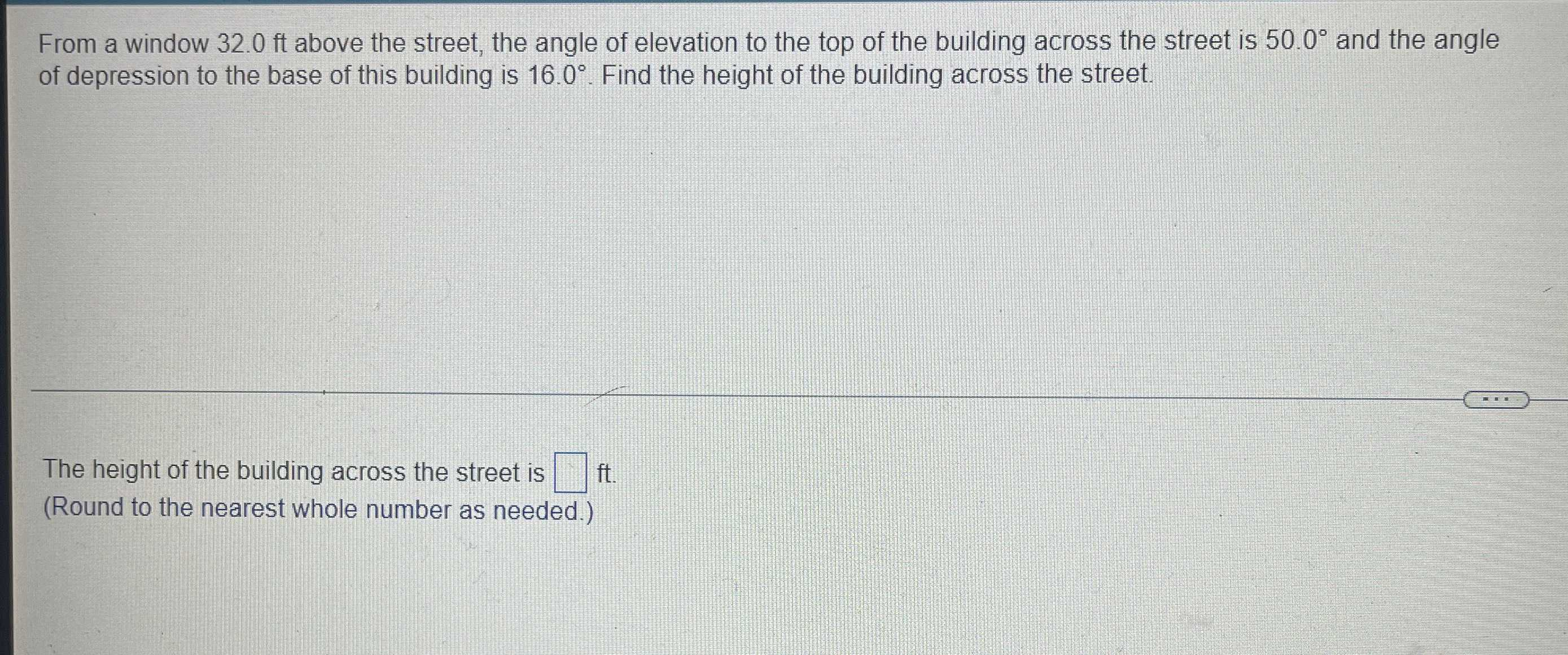### Still have math questions?

Trigonometry
QuestionFrom a window $$32.0 ft$$ above the street, the angle of elevation to the top of the building across the street is $$50.0 ^ { \circ }$$ and the angle of depression to the base of this building is $$16.0 ^ { \circ }$$ .

Find the height of the building across the street.

The height of the building across the street is _____ft.

(Round to the nearest whole number as needed.)

The height (h)(h) of the building across the street is:

$$h= h_1+ 32$$ft              (Eq. 1)

Use the tangent of the angle of elevation ($$θ_e= 50°$$) to find $$h_1$$, where the opposite side is$$h_1$$ and the adjacent side is x:

$$tanθ_e= \frac{opposite \space side}{adjacent\space side}$$

$$tan50° = \frac{h_1}{x}$$

$$x \space tan50° = h_1$$

$$h_1= x\space tan50°$$    (Cross multiply x)       (Eq. 2)

Substituting Eq. 2 in Eq. 1:

$$h= h_1+ 32$$ ft            (Eq. 1)

$$h= x\space tan50° + 30$$   ft (Eq. 3)

Now we need to know the length of x, so use the tangent of the angle of depression $$(θ_d= 20\degree)$$ to look for it. Using the height of the window of 32 ft as the opposite side and x as the adjacent side, solve for x

$$tanθ_d= \frac{opposite \space side}{adjacent\space side}$$

$$tan20\degree= \frac{32}{x}$$

$$x\space tan20\degree= 32$$

$$x= \frac{32}{tan20\degree}$$

x=$$\frac{32}{0.3639702343}$$

x=87.9192774144

The value of x remains unrounded because this is not yet the final answer. Substituting this in Eq. 3, the height of the building is:

$$h= x\space tan50\degree+ 32 ft$$

$$h= 87.9192774144\space tan50\degree+ 32$$ ft

=104.77810+32 ft

=136.7781

≈136.8 ft

Thus, the building across the street is 136.8 feet high.

Solution
View full explanation on CameraMath App.